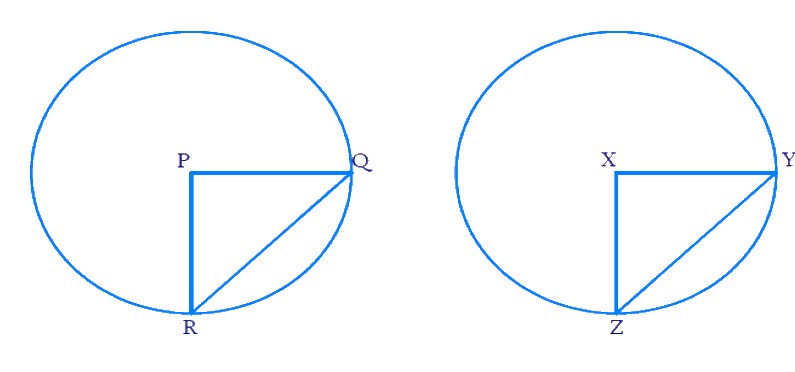# Exercise E10.2 Circles NCERT Solutions Class 9

Go back to  'Circles'

## Chapter 10 Ex.10.2 Question 1

Recall that two circles are congruent if they have the same radii. Prove that equal chords of congruent circles subtend equal angles at their centers.

### Solution

What is known?

Two circles are congruent if they have same radii.

What is unknown?

Equal chords of congruent circles subtend equal angles at their centers.

Reasoning:

Using chords are equal and the fact that circles are congruent, we prove the statement using Side-Side-Side (SSS criteria) and Corresponding parts of congruent triangles (CPCT).

Steps:Let \begin{align} {QR} \end{align} and \begin{align} {YZ} \end{align} be the equal chords of $$2$$ congruent circles.

\begin{align} {QR = YZ} \end{align}

We need to prove that they subtend equal angles at centre. i.e. \begin{align}\angle{QPR} =\angle {YXZ}\end{align}

We know that the radii of both the circles are equal. So we get:

\begin{align} {PR = PQ = XZ = XY} \end{align}

Consider the $$2$$ triangles \begin{align} \Delta { PQR} \end{align} and \begin{align} \Delta { XYZ} \end{align}.

\begin{align} &{PQ = XY} \qquad \text{(Radii are equal)}\\&{PR = XZ} \qquad \text{(Radii are equal)}\\&{QR = YZ} \qquad \text{(Chords are equal)}\end{align}

By SSS criteria, \begin{align} \Delta\,{PQR}\end{align} is congruent to \begin{align} \Delta\;{XYZ}.\end{align}

So by CPCT (Corresponding parts of congruent triangles) we get \begin{align} \angle{QPR}=\angle{YXZ}.\end{align}

Hence proved that equal chords of congruent circles subtend equal angles at their centres.

## Chapter 10 Ex.10.2 Question 2

Prove that if chords of congruent circles subtend equal angles at their centers, then the chords are equal.

### Solution

What is known?

Two circles are congruent, and their chords subtend equal angles at their centers.

What is unknown?

To find whether the chords are equal.

Reasoning:

Using equal angles at centres and the fact that circles are congruent, we prove the statement using Side-Angle-Side (SAS criteria) and Corresponding parts of congruent triangles (CPCT).

Steps:Draw chords \begin{align} {QR}\end{align} and \begin{align} {YZ}\end{align} in $$2$$ congruent circles respectively. Join the radii \begin{align} {PR}, \; {PQ} \end{align} and \begin{align} {XY, XZ }\end{align} respectively.

Given that chords subtend equal angles at centre. So\begin{align}\angle {QPR}=\angle {YXZ}\,.\end{align}

We need to prove that chords are equal. i.e \begin{align}{QR = YZ}.\end{align}

Since the circles are congruent, their radii will be equal.

\begin{align}{PR = PQ = XZ = XY}\end{align}

Consider the $$2$$ triangles \begin{align} \Delta\,{PQR}\end{align} and \begin{align} \Delta\,{XYZ}.\end{align}

\begin{align}{{PQ}} & ={{XY}} \qquad \;\; \begin{pmatrix} \text { Radii } \\ \text{are equal }\end{pmatrix} \\ {\angle {QPR}} &={\angle {YXZ}} \quad \begin{pmatrix} \! \text { Chords } \! \\ \! \text{subtend} \! \\ \! \text{equal angles} \! \\ \! \text{ at centre } \! \end{pmatrix} \\ {{PR}} &= {{XZ}} \qquad \;\; \begin{pmatrix} \text { Radii } \\ \text{are equal }\end{pmatrix} \end{align}

By SAS criteria \begin{align} \Delta { PQR}\end{align} is congruent to \begin{align} \Delta { XYZ.}\end{align}

So by CPCT (Corresponding parts of congruent triangles) \begin{align} {QR = YZ.}\end{align}

Hence proved if chords of congruent circles subtend equal angles at their centres then the chords are equal.

Related Sections
Related Sections
Instant doubt clearing with Cuemath Advanced Math Program